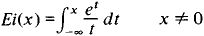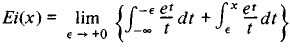Exponential Integral

Also found in: Wikipedia.

exponential integral

[‚ek·spə′nen·chəl ′int·ə·grəl]
(mathematics)
The function defined to be the integral from x to ∞ of (e -t / t) dt for x positive.
McGraw-Hill Dictionary of Scientific & Technical Terms, 6E, Copyright © 2003 by The McGraw-Hill Companies, Inc.
The following article is from The Great Soviet Encyclopedia (1979). It might be outdated or ideologically biased.

Exponential Integral

a special function defined by the integralThis integral cannot be expressed in closed form through elementary functions. If x > 0, then the integral is understood in the sense of its principal value:Mentioned in ?
References in periodicals archive ?
where E[n,z] = [[integral].sup.[infinity].sub.1] [t.sup.-n] [e.sup.-zt] is 'exponential integral function'.
However, the incomplete gamma function is a more complete way to calculate the temperature integral than the exponential integral because [p.sub.m](x) = [LAMBDA](-(m + 1),x) yielded consistent results (low errors) for any m, integer or non-integer in the interval 1 [less than or equal to] x [less than or equal to] 100 tested in the supplementary material.
Among the topics are complete elliptic integrals, the Riemann zeta function, some automatic proofs, the error function, hyper-geometric functions, Bessel-K functions, polylogarithm functions, evaluation by series, the exponential integral, confluent hyper-geometric and Whittaker functions, and the evaluation of entries in Gradshteyn and Ryzhik employing the method of brackets.
where [[bar.[gamma]].sub.bm] = [P.sub.b]/[[sigma].sup.2.sub.m] and [E.sub.1] (x) = [[integral].sup.[infinity].sub.x]([e.sup.-t]/t)dt is the exponential integral function.
A.1 Mathematical tools: Exponential integral, sine integral and cosine integral
For indefinite integrals, an extended version of the Risch code is used whenever both the integrand and integral can be expressed in terms of elementary functions, exponential integral functions, polylogarithms and other related functions.
and ExpIntegral E[n, z] gives the exponential integral function [E.sub.n] (z), which is defined by
Exponential Integral, Logarithmic Integral, Sine and Cosine Integrals
In Section 2, we provide an explicit expression of the moment generating function in terms of the exponential integral function.
We define, for x > 0, the function F(x) = x[e.sup.x][E.sub.1](x), where [E.sub.1] (x) denotes the exponential integral (, (5.1.1)).
Uniform convergence and computation of the generalized exponential integrals. J.

Site: Follow: Share:
Open / Close